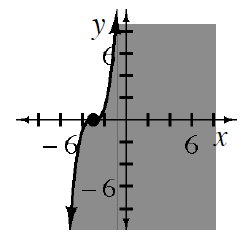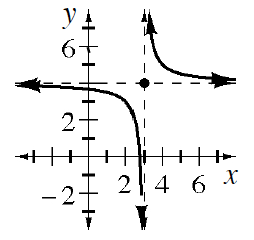Home > CCA2 > Chapter 4 > Lesson 4.2.4 > Problem4-102

4-102.

On separate pairs of axes, sketch the graph of each equation or inequality below. Homework Help ✎

1. $y+5=(x−2)^2$

1. $y\le(x+3)^3$1. $y = 4 + \frac { 1 } { x - 3 }$